# MA2219

•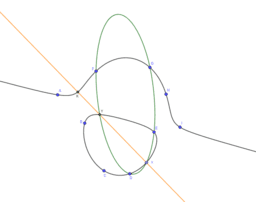### Coresidual of four points on a cubic

Activity

MA2219

•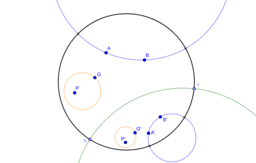### Rigid motions in the Poincare disk

Activity

MA2219

•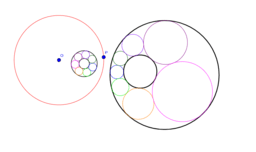### Steiner's Porism Example

Activity

MA2219

•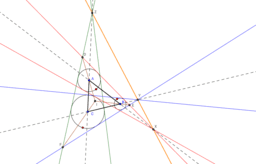### Proof of Monge's theorem using Desargues' theorem

Activity

MA2219

•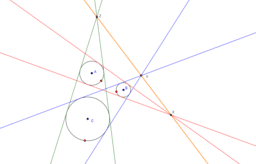### Monge's theorem

Activity

MA2219

•### Monge's theorem

Activity

MA2219

•### Another proof of Pythagoras' Theorem

Activity

MA2219

•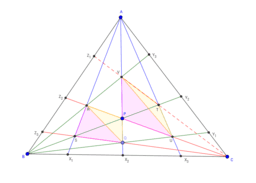### Hint for Desargues' theorem proof

Activity

MA2219

•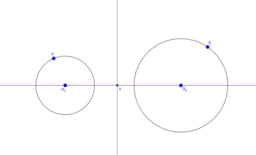### Construct radical axis of two circles

Activity

MA2219

•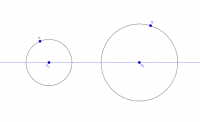### Exercise: Construct radical axis of two circles

Activity

MA2219

•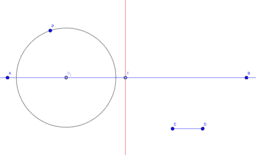### Exercise: Construct circles in the pencil given by a circle and the radical axis

Activity

MA2219

•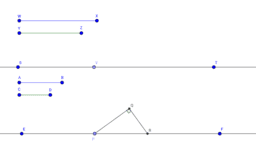### Construct a right-angled triangle with hypotenuse on a given line

Activity

MA2219

•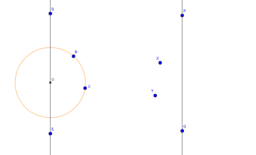### Construct a circle with centre on a given line and passing through two points

Activity

MA2219

•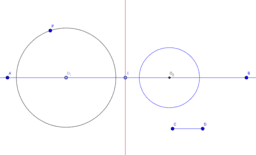### Construct circles in the pencil given by a circle and the radical axis

Activity

MA2219

•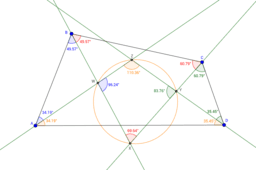### The angle bisectors of a quadrilateral form a cyclic quadrilateral

Activity

MA2219

•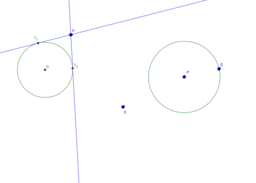### Construct tangent to circle

Activity

MA2219

•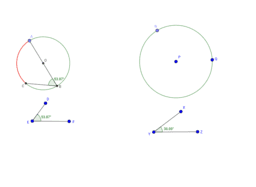### Construct a segment subtending a given angle

Activity

MA2219

•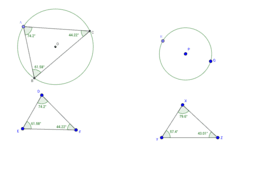### Constructing a triangle in a circle similar to a given triangle

Activity

MA2219

•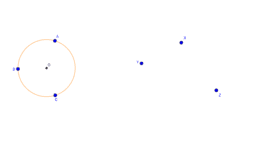### Constructing the centre of a circle through three points

Activity

MA2219

•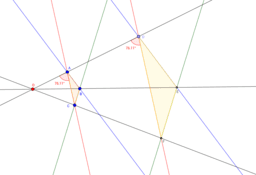### Desargues' theorem for parallel lines

Activity

MA2219

•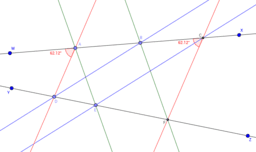### Pappus' theorem for parallel lines

Activity

MA2219

•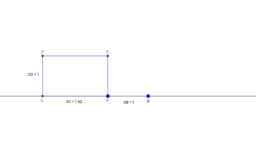### Constructing the golden ratio

Activity

MA2219

•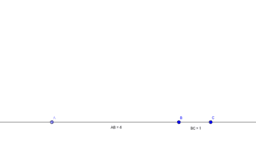### Constructing the square root of a number

Activity

MA2219

•Activity

MA2219